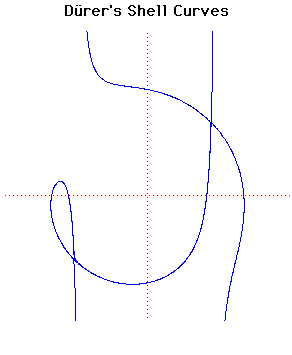# Durer's Shell CurvesCartesian equation:
(x2 + xy + ax - b2)2 = (b2 - x2)(x - y + a)2

These curves appear in Dürer's work Instruction in measurement with compasses and straight edge(1525).

Dürer calls the curve 'ein muschellini' which means a conchoid, but since it is not a true conchoid we have called it Dürer's shell curve (muschellini = conchoid = shell).

This curve arose from Dürer's work on perspective. He constructed the curve in the following way. He drew lines QRP and P'QR of length 16 units through Q (q, 0) and R (0, r) where q + r = 13. The locus of P and P' as Q and R move on the axes is the curve. Dürer only found one of the two branches of the curve.

The envelope of the line P'QRP is a parabola and the curve is therefore a glissette of a point on a line segment sliding between a parabola and one of its tangents.

There are a number of interesting special cases:
In the above formula we have:

b = 0 : Curve becomes two coincident straight lines x2 = 0.

a = 0 : Curve becomes the line pair x = b/√2, x = -b/√2
together with the circle x2 + y2 = b2.

a = b/2 : The curve has a cusp at (-2a, a).

JOC/EFR/BS January 1997